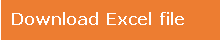# Extract Specific Color Index using VBA

In case you want to copy a particular formatted cell or colored cells from one column to the next column then this article is for you. In this article, we will learn how to copy colored cells using VBA code.

Following is the snapshot of the data we have i.e. column A contains data with few yellow colored cells.

To extract the data that is highlighted in yellow color only; we need to create a UDF to show the colored cells only in column B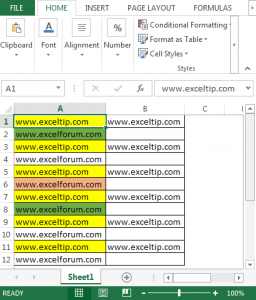• To create User Defined Function, we will follow the below steps:
• Click on Developer tab
• From Code group, select Visual Basic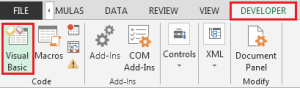• We are required to copy below code in the standard module

Function IsYellow(ByRef r As Range)

If r.Interior.ColorIndex = 6 Then

IsYellow = r.Value

Else

IsYellow = vbNullString

End If

End Function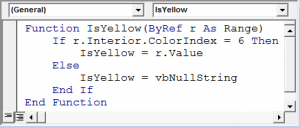• In cell B1, the formula will be =IsYellow(A1)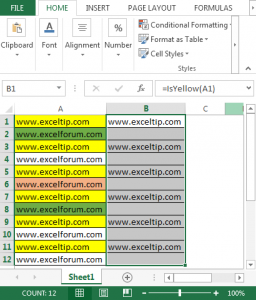The above code is specifically matching the color# 6 which is meant for Yellow color only. Hence, the udf will extract only the values that contain color#6.

In case we want to extract red color cells then we need to check the designated color number & simply replace with yellow color number.

In this way, we can retrieve the yellow font color cells using vba code.